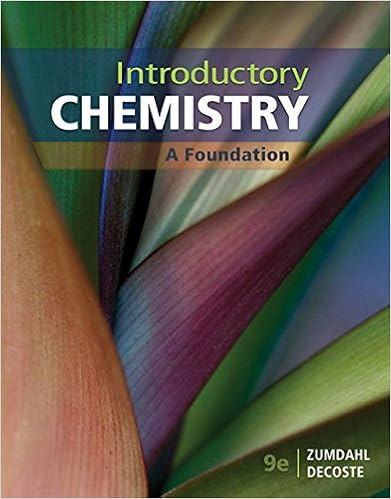# If e a for a certain biological reaction is 50 kjmol

• Notes
• 22
• 100% (2) 2 out of 2 people found this document helpful

This preview shows page 21 - 22 out of 22 pages.

##### We have textbook solutions for you!
The document you are viewing contains questions related to this textbook.The document you are viewing contains questions related to this textbook.
Chapter 6 / Exercise 16
Introductory Chemistry: A Foundation
ZumdahlExpert Verified
79.If Eafor a certain biological reaction is 50. kJ/mol, by what factor (how many times will the rate of this reaction increase when body temperature increases from 37°C (normal) to 40°C (fever)? A. 2.0B.2.0 ×10C. 1.0002D. 1.15E.1.20
5
80.For the overall chemical reaction shown below, which one of the following statements can be rightly assumed?2H2S(g) + O2(g) 2S(s) + 2H2O(l)
81.For the reaction whose rate law is rate = k[X], a plot of which of the following is a straight line?
21
##### We have textbook solutions for you!
The document you are viewing contains questions related to this textbook.The document you are viewing contains questions related to this textbook.
Chapter 6 / Exercise 16
Introductory Chemistry: A Foundation
ZumdahlExpert Verified
82.Calculate the activation energy, in kJ/mol, for the redox reactionSn2++ 2Co3+Sn4++ 2Co2+.
83.In which of the forms listed below would 0.5 g aluminum react the fastest with gaseous chlorine at 25°C? A.0.5 g aluminum divided into 1,000 piecesB.0.5 g aluminum in one pieceC.0.5 g aluminum divided into 100 piecesD.All the choices will react at the same rate since the temperature is the same.E.0.5 g aluminum divided into 10 pieces
22
•••